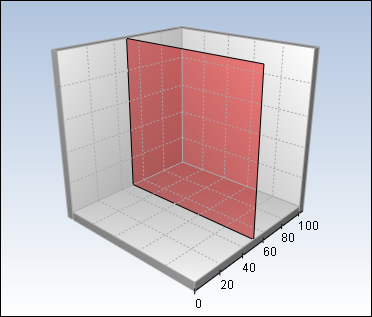﻿ Converting Chart Coordinates
Nevron .NET Vision
Chart for .NET / User's Guide / Chart / Converting Chart Coordinates

In This Topic
Converting Chart Coordinates
In This Topic

It is a common task to convert from different chart coordinates - for example if you want to locate a data point which is closest to the mouse cursor or to position a watermark over an axis value. This topic shows how to convert from scale to model, from model to viewport and vice versa.

The first thing you should know about coordinate transformations is that you can use them only after the chart is rendered or during rendering itself. This ensures that the axes and control viewport have been properly calculated. The examples shipped with the control perform coordinate transformations in the chart AfterPaint and OnMouseDown events.

Converting from Scale to Model Coordinates

Scale coordinates are the coordinates of the data points relative to the chart axes. Each data point translates to [x, y, z] scale coordinate which are then scaled from the chart axes to model coordinates. Therefore in order to convert a scale coordinate to model coordinate you need to know the scale axis. The TransformScaleToModel function of the axis converts a scale coordinate to model coordinate. It accepts two parameters - the first one specifies whether to clamp the scale coordinate to the axis ruler and the second one is the scale coordinate itself. The following code locates the model coordinates corresponding to the origin of the scale coordinate system:

C#
Copy Code
```NCartesianChart chart = chartControl.Charts;
NVector3DF vecModelPoint = new NVector3DF();

vecModelPoint.X = chart.Axis(StandardAxis.PrimaryX).TransformScaleToModel(false, 0);
vecModelPoint.Y = chart.Axis(StandardAxis.PrimaryY).TransformScaleToModel(false, 0);
vecModelPoint.Z = chart.Axis(StandardAxis.Depth).TransformScaleToModel(false, 0);
```
Visual Basic
Copy Code
```Dim chart As NCartesianChart = chartControl.Charts(0)
Dim vecModelPoint As New NVector3DF

vecModelPoint.x = chart.Axis(StandardAxis.PrimaryX).TransformScaleToModel(False, 0)
vecModelPoint.y = chart.Axis(StandardAxis.PrimaryY).TransformScaleToModel(False, 0)
vecModelPoint.z = chart.Axis(StandardAxis.Depth).TransformScaleToModel(False, 0)
```
Converting from Model to Viewport and Control Client Coordinates

These are probably one of the most useful conversions because they allow you to position custom drawings in the control canvas according to chart model coordinates. The control client coordinates are coordinates relative to the control origin in the form. The control viewport coordinates are coordinates relative to the control origin in the form, but with applied offset depending on the current background frame. You use the TransformModelToClient and TransformModelToViewport functions of the NChart object to perform these conversions respectively. The following code shows how to place a watermark at the origin of the control model coordinate system:

C#
Copy Code
```NWatermark watermark = chartControl.Watermarks;
NCartesianChart chart = chartControl.Charts;

NVector3DF vecModelPoint = new NVector3DF();

if (chart.TransformModelToViewport(chartControl.View.Context, vecModelPoint, ref vecViewPoint))
{
watermark.Location = new NPointL( new NLength(vecViewPoint.X, NGraphicsUnit.Pixel), new NLength(vecViewPoint.Y, NGraphicsUnit.Pixel));
}
```
Visual Basic
Copy Code
```Dim watermark As NWatermark = chartControl.Watermarks(0)
Dim chart As NCartesianChart = chartControl.Charts(0)

Dim vecModelPoint As New NVector3DF

If (chart.TransformModelToViewport(chartControl.View.Context, vecModelPoint, vecViewPoint) = True)Then
watermark.Location = New NPointL(New NLength(vecViewPoint.X, NGraphicsUnit.Pixel), New NLength(vecViewPoint.Y, NGraphicsUnit.Pixel))
End If
```
Converting from Control Client to Model Coordinates

Converting from 2D to 3D coordinates is somewhat more complex than 3D to 2D because you need to recover a "missing coordinate". Therefore you need a plane in 3D which recovers the coordinate lost from the 3D to 2D conversion. You use the TransformClientToModel function of the chart to perform this conversion. It has the following definition:

C#
Copy Code
```public bool TransformClientToModel(NChartRenderingContext context, NAxis horzAxis, NAxis vertAxis, NAxis depthAxis, float fDepthValue, Point ptViewPoint, ref NVector3DF vecModelPoint)
```
Visual Basic
Copy Code
```Public Function TransformClientToModel(ByVal context As NChartRenderingContext, ByVal horzAxis As NAxis, ByVal vertAxis As NAxis, ByVal depthAxis As NAxis, ByVal fDepthValue As Single, ByVal ptViewPoint As Point, ByRef vecModelPoint As NVector3DF) As Boolean
```

The first parameter defines the rendering context. Usually this is the Context property of the NView class which is used in View property of the chartControl. The second and the third parameters define the plane normal vector which is orthogonal to the plane formed from the horzAxis and vertAxis axes. The depthAxis and fDepthValue parameters define a point in 3D which lies on the plane. The following picture shows how this plane looks like in the case of the primaryX, primaryY and primaryZ axes:In this case the depth value is set to 50. Now when you try to convert a client point that lies on the red area the converted model point will have the [x, y, z] coordinates of the point which lies on the model plane.
Converting from Model to Scale Coordinates

Finally you can convert model coordinates to scale coordinates by using the TransformModelToScale function of the axes. The following code gets the scale coordinates of the mouse cursor coordinates mapped on the XY plane at depth value 50:

C#
Copy Code
```private void OnMouseDown(object sender, MouseEventArgs e)
{
NChart chart = chartControl.Charts;
Point ptViewPoint = new Point(e.X, e.Y);
float fDepthValue = 50.0f;

// XY plane
NAxis horzAxis = chart.Axis(StandardAxis.PrimaryX);
NAxis vertAxis = chart.Axis(StandardAxis.PrimaryY);
NAxis depthAxis = chart.Axis(StandardAxis.Depth);

NVector3DF vecModelPoint = new NVector3DF();

if (chart.TransformClientToModel(chartControl.View.Context, horzAxis, vertAxis, depthAxis, fDepthValue, ptViewPoint, ref vecModelPoint))
{
double fX = horzAxis.TransformModelToScale(vecModelPoint.X, true);
double fY = vertAxis.TransformModelToScale(vecModelPoint.Y, true);
double fZ = depthAxis.TransformModelToScale(vecModelPoint.Z, true);

// do something with the fX, fY and fZ scale coordinates
}
}
```
Visual Basic
Copy Code
```Private Sub OnMouseDown(ByVal sender As System.Object, ByVal e As MouseEventArgs)
Dim chart As NCartesianChart = chartControl.Charts(0)
Dim ptViewPoint As New Point(e.X, e.Y)
Dim fDepthValue As Single = 50.0F

' XY plane
Dim horzAxis As NAxis = chart.Axis(StandardAxis.PrimaryX)
Dim vertAxis As NAxis = chart.Axis(StandardAxis.PrimaryY)
Dim depthAxis As NAxis = chart.Axis(StandardAxis.Depth)

Dim vecModelPoint As New NVector3DF

If (chart.TransformClientToModel(chartControl.View.Context, horzAxis, vertAxis, depthAxis, fDepthValue, ptViewPoint, vecModelPoint)) Then
Dim fX As Double = horzAxis.TransformModelToScale(vecModelPoint.X, True)
Dim fY As Double = vertAxis.TransformModelToScale(vecModelPoint.Y, True)
Dim fZ As Double = depthAxis.TransformModelToScale(vecModelPoint.Z, True)

'do something with the fX, fY and fZ scale coordinates
End If
End Sub
```
Related Examples

Windows forms: Coordinate Transformations\Scale to Viewport

Windows forms: Coordinate Transformations\Viewport to Scale